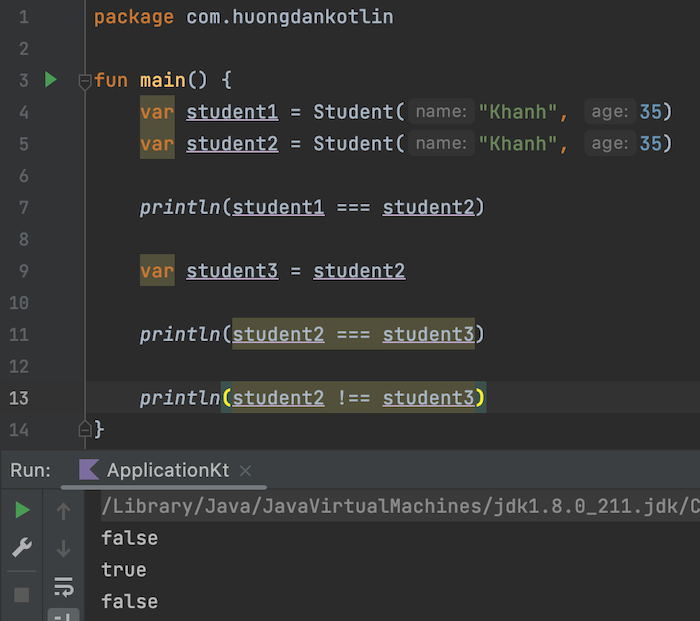# Equality in Kotlin

In Java, when comparing whether 2 objects initialized from a class are equal or not, we need to implement 2 equals() and hashCode() methods for that class and will use the equals() method to compare them.

For example, I have a Student class containing name and age information, defined with equals() and hashCode() methods as follows:

To compare objects of Student class, we need to use equals() method instead of the equality operator, for example as follows:

Result: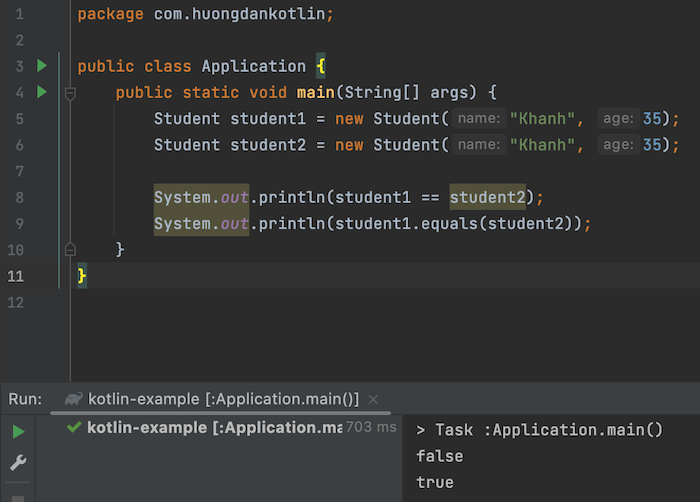As you can see, with the equality operator, comparing two Student objects will return false.

However, in Kotlin, if you write the same code as above:

You will see the following output when you run this code: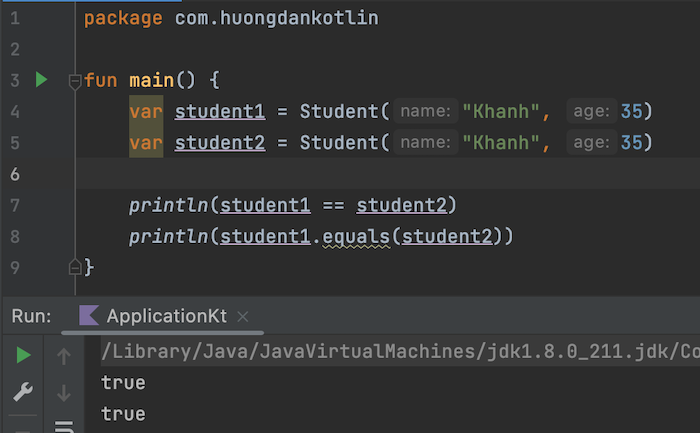Obviously, there is a difference here between Java and Kotlin right? Using the equality operator to compare 2 objects of Student class with the same name and same age in Kotlin still returns true. That’s because, the equality operator in Kotlin is structural equality, as long as 2 objects have the same content, no matter the address of these objects in memory, they will be the same. For this reason, if you use the equals() method to compare two objects in Kotlin, IntelliJ will suggest you use the equality operator to make your code more concise.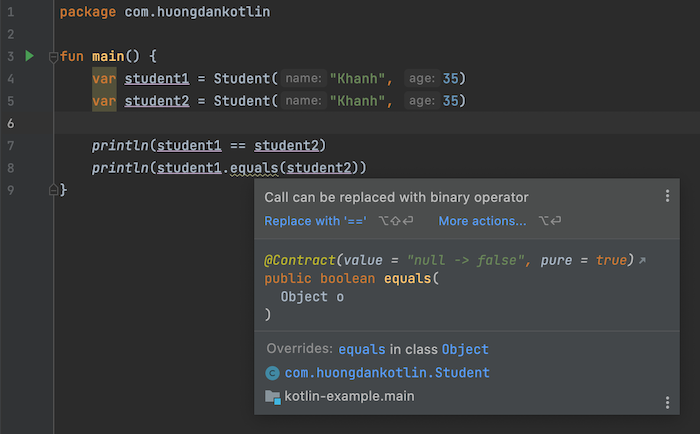To compare reference (referential equality) in Kotlin, you need to write code to compare 2 objects with triple equals as follows:

hen, the result when comparing will be the same as Java, always false: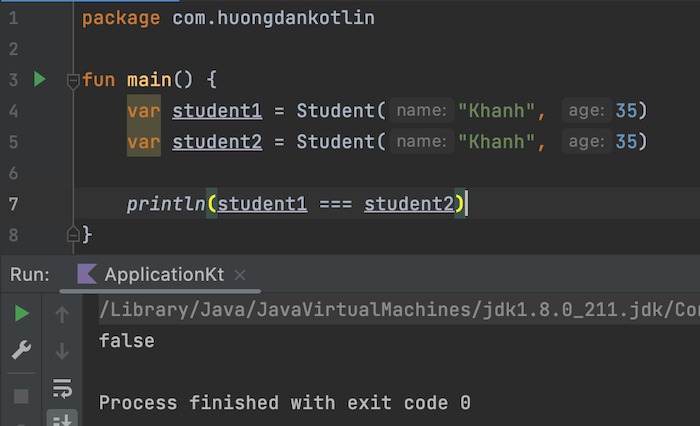If:

the result of the last line of code will be true: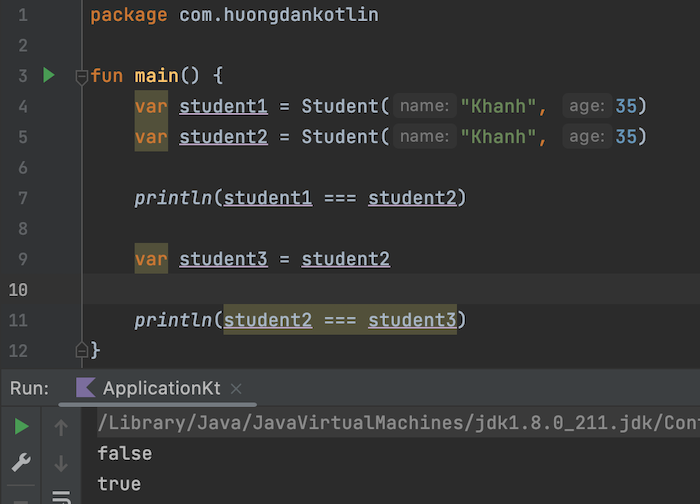We have equality comparison and non-equal comparison in Java with “==” and  “!=”. In Kotlin we use “===” and “!==”, for example like this:

Result: